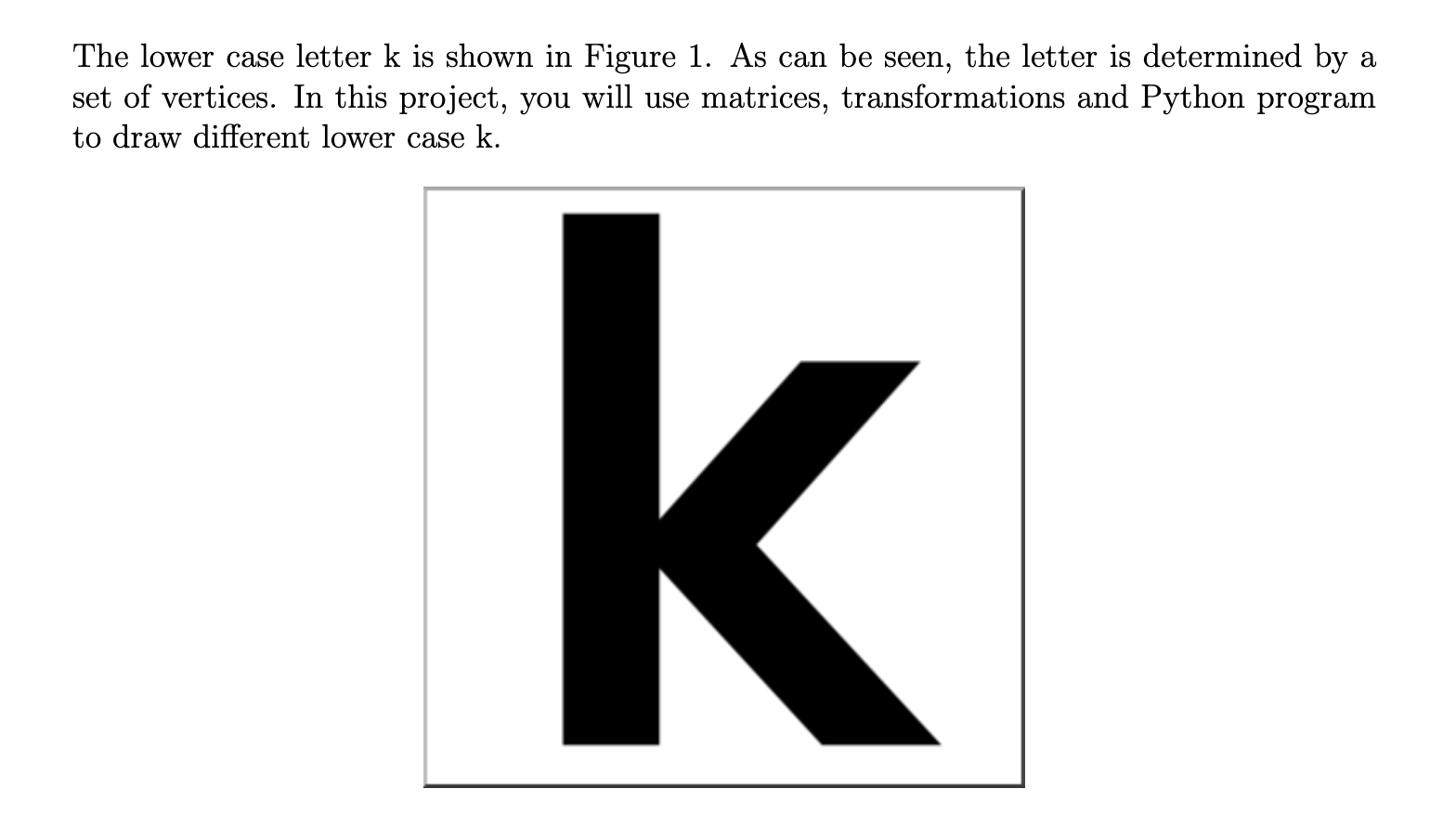# Question 1. Design vertices of the lower case k. 2. What is the adjacency matrix? 3. Using Python program to draw the lower case k. 4. Rotate the lower case k by 45◦counterclockwise. 5. Draw the backward lower case k. The lower case letter k is shown in Figure 1. As can be seen, the letter is determined by a set of vertices. In this project, you will use matrices, transformations and Python program to draw different lower case k. k.RBGWGG The Asker · Computer Science1. Design vertices of the lower case k.
2. What is the adjacency matrix?
3. Using Python program to draw the lower case k.
4. Rotate the lower case k by 45◦counterclockwise.
5. Draw the backward lower case k.

Transcribed Image Text: The lower case letter k is shown in Figure 1. As can be seen, the letter is determined by a set of vertices. In this project, you will use matrices, transformations and Python program to draw different lower case k. k.
More
Transcribed Image Text: The lower case letter k is shown in Figure 1. As can be seen, the letter is determined by a set of vertices. In this project, you will use matrices, transformations and Python program to draw different lower case k. k.